TitleCollege Algebra
Answer/Discussion to Practice Problems
Tutorial 22: Linear Inequalities

WTAMU > Virtual Math Lab > College Algebra > Tutorial 22: Linear InequalitiesAnswer/Discussion to 1a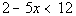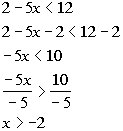Interval notation: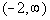Graph: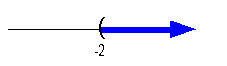*Inv. of add. 2 is sub. 2   *Inv. of mult. by -5 is div. both sides by -5, so reverse inequality sign   *Open interval indicating all values greater than -2   *Visual showing all numbers greater than -2 on the number line

 Interval notation: We have an open interval since we are not including where it is equal to -2.   x is greater than -2, so -2 is our smallest value of the interval so it goes on the left.  Since there is no upper endpoint (it is ALL values greater than -2), we put the infinity symbol on the right side.  The curved end on -2 indicates an open interval.  Infinity always has a curved end because there is not an endpoint on that side. Graph:  We use the same type of notation on the endpoint as we did in the interval notation,  a curved end.  Since we needed to indicate all values greater than -2, the part of the number line that was to the right of -2 was darkened.Answer/Discussion to 1b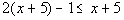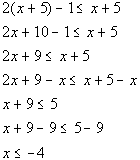Interval notation: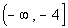Graph: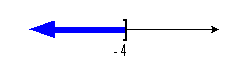*Distributive property *Get x terms on one side, constants on the other side *Inv. of add. 9 is sub. by 9       *Closed interval indicating all values less than or = to -4   *Visual showing all numbers less than or = to -4 on the number line

 Interval notation: We have a closed interval since we are including where it is equal to -4.   This time  x is less than or equal to -4, so -4 is our largest value of the interval so it goes on the right.  Since there is no lower endpoint (it is ALL values less than or equal to -4), we put the negative infinity symbol on the left side.  The boxed end on -4 indicates a closed interval.  Negative infinity always has a curved end because there is not an endpoint on that side. Graph:  Again, we use the same type of notation on the endpoint as we did in the interval notation, a boxed end.  Since we needed to indicate all values less than or equal to -4, the part of the number line that was to the left of -4 was darkened.Answer/Discussion to 1c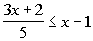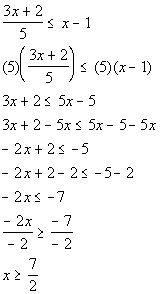Interval notation: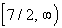Graph: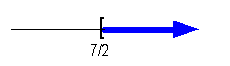*Mult. both sides by LCD of 5     *Get x terms on one side, constants on the other side   *Inv. of add. 2 is sub. by 2 *Inv. of mult. by -2 is div. both sides by -2, so reverse inequality sign         *Closed interval indicating all values greater than or equal to 7/2   *Visual showing all numbers greater than or equal to 7/2 on the number line

 Interval notation: This time we have a closed interval since we are including where it is equal to 7/2.   x is greater than or equal to 7/2, so 7/2 is our smallest value of the interval so it goes on the left.  Since there is no upper endpoint (it is ALL values greater than or equal to 7/2), we put the infinity symbol on the right side.  The boxed end on 7/2 indicates a closed interval.  Infinity always has a curved end because there is not an endpoint on that side. Graph:  Again, we use the same type of notation on the endpoint as we did in the interval notation,  a boxed end this time.  Since we needed to indicate all values greater than or equal to 7/2, the part of the number line that was to the right of 7/2 was darkened.Answer/Discussion to 2a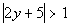The absolute value expression is already isolated.

 Step 2:  Use the definition of absolute value to set up the inequality without absolute values. AND Step 3:  Solve the linear inequalities set up in step 2.

 The distance that the expression 2y + 5 is away from the origin needs to be greater than or equal to 1.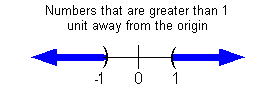All numbers that are less than -1 OR greater than 1 are greater than 1 unit away from the origin.  So the expression 2y + 5 needs to be less than -1 OR greater than 1.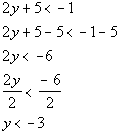OR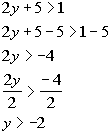Interval notation: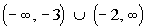Graph: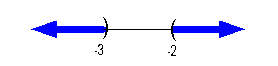*First inequality, where it is less than -1 *Inv. of add. 5 is sub. 5 *Inv. of mult. by 2 is div. by 2             *Second inequality, where it is greater than 1 *Inv. of add. 5 is sub. 5 *Inv. of mult. by 2 is div. by 2           *All values less than -3 or greater than -2   *Visual showing all numbers less than -3 or greater than -2

 Interval notation: This time we have two open intervals since we are not including the endpoints -3 and -2.  In the first interval, y is less than -3, so -3 is our largest value of the interval so it goes on the right.  Since there is no lower endpoint of that first interval, we put negative infinity on the left side.  The curved end on -3 indicates an open interval.  Infinity always has a curved end because there is not an endpoint on that side.  In the second interval,  y is greater than -2, so -2 is our smallest value of the interval so it goes on the left.  Since there is no upper endpoint of that second interval, we put the infinity symbol on the right side.  The curved end on -2 indicates an open interval.  Infinity always has a curved end because there is not an endpoint on that side. Graph:  Again, we use the same type of notation on the endpoints as we did in the interval notation,  a curved end on both y = -3 and y = -2.  Since we needed to indicate all values less than -3 OR greater than -2, the parts of the number line that are to the left of -3 and to the right of -2 were darkened.Answer/Discussion to 2b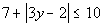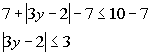*Inv. of add. 7 is sub. 7 *Abs. value exp. isolated

 Step 2:  Use the definition of absolute value to set up the inequality without absolute values. AND Step 3:  Solve the linear inequalities set up in step 2.

 The distance that the expression 3y - 2 is away from the origin needs to be less than 3.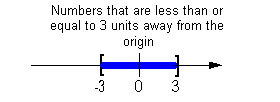All numbers between -3 and 3 are less than 3 units away from the origin.  So, the expression 3y - 2 needs to be between -3 and 3.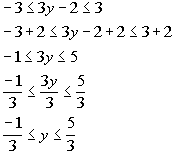Interval notation: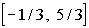Graph: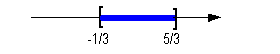*Inv. of sub. 2 is add. 2 *Apply steps to all three parts  *Inv. of mult. by 3 is div by 3 *Apply steps to all three parts        *Closed interval indicating all values between -1/3 and 5/3, inclusive   *Visual showing all numbers between -1/3 and 5/3, inclusive

 Interval notation: This time we have a closed interval since we are including both endpoints. y is between -1/3 and 5/3, inclusive, so -1/3 is our smallest value of the interval so it goes on the left and 5/3 goes on the right.   The boxed end on both numbers indicate a closed interval on both sides.  Graph:  Again, we use the same type of notation on the endpoints as we did in the interval notation,  a boxed end on both ends.  Since we needed to indicate all values between -1/3 and 5/3,  the part of the number line that is in between -1/3 and 5/3 was darkened.Answer/Discussion to 2c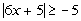The absolute value expression is already isolated.

 Step 2:  Use the definition of absolute value to set up the inequality without absolute values. AND Step 3:  Solve the linear inequalities set up in step 2.

 Again, be careful - since the absolute value (the left side) is always positive, and positive values are always greater than negative values, the answer is all real numbers.  No matter what value you plug in for x, when you take the absolute value the left side will be positive.  All positive numbers are greater than -5.Answer/Discussion to 2dThe absolute value expression is already isolated.

 Step 2:  Use the definition of absolute value to set up the inequality without absolute values. AND Step 3:  Solve the linear inequalities set up in step 2.

 Be careful, since the absolute value (the left side) is always positive, and positive values are always greater than negative values, the answer is no solution.  There is no value that we can put in for x that would make this inequality true.

Last revised on Dec. 29, 2009 by Kim Seward.
All contents copyright (C) 2002 - 2010, WTAMU and Kim Seward. All rights reserved.